## 本人写的一个C＋＋小银行系统，很多错误，牛牛们给指正一下。

intruder 发布于 2012/04/10 08:32

为什么80%的码农都做不了架构师？->>>1.BANK.H --This is head file.

```// bink.h -- a bank-system program
#ifndef BANK_H_
#define BANK_H_

class Bank
{
private:
enum {LENGTH = 40};
char name[LENGTH];
char account_number[LENGTH];
unsigned long int share;
unsigned long long sum_shares;
public:
Bank(char *, char *, int);
~Bank();
unsigned long int into_money(unsigned long int money);
unsigned long int out_money(unsigned long int money);
void show_info()const;
};

#endif```

2.BANK.CPP ---This is the define of head file

```// bank.cpp -- to decaleration the bank.h define
#include <iostream>
#include "bank.h"

Bank::Bank(char *, char *, int)
{
share = 5;
sum_shares = share;
}

Bank::~Bank()
{
}

unsigned long int Bank::into_money(unsigned long int money)
{
using std::cout;
if (money < 0)
{
cout << "You can't do this!\n"
<< "Please try again!\n";
}
else
{
share = money;
sum_shares += share;
}
show_info();
return sum_shares;
}

unsigned long int Bank::out_money(unsigned long int money)
{
using std::cout;
if (money < 0)
{
cout << "You can't do this!\n"
<< "Please try again!\n";
}
else if (money > sum_shares)
{
cout << "You don't have enough money to out!\n"
<< "Please try again!\n";
}
else
{
share = money;
sum_shares -= share;
}
show_info();
return sum_shares;
}
void Bank::show_info()const
{
using std::cout;
using std::endl;

cout << "You information:" << endl;
cout << "Account Name:# " << name << endl
<< "Account Number:# " << account_number << endl
<< "You have : \$" << sum_shares << " int the bank."
<< endl;
cout << "Thank for your use" << endl << "Bye.\n";
}```

3.Main function -- Running with here

```// banker.cpp -- the main file in the bank-system program
#include <iostream>
#include "bank.h"

int main()
{
using std::cout;
using std::cin;
using std::endl;
cout << "Please login your bank information about:\n \"Accout Name\"-\"Accout Number\"and How much money do you want to in:";
cout << endl;
cout << "Of course ,you must create a Accout before you do this ...." << endl;
Bank person = Bank("Zhouc", "1234567",100);
unsigned long int number;
int which;
cout << "Please choose your want:" << endl;
cout << "1.into the money" << endl;
cout << "2.out the money" << endl;
cout << "3.show your have!" << endl;
cout << "4.And 'Q' or 'q' to quit! " << endl;
while (cin >> which && (cin.get() != 'Q' || cin.get() != 'q'))
{
while (cin.get() != '\n')
continue;
switch(which)
{
case "1":cout << "Please input the shares of money:\n";
cin >> number;
person.into_money(number);
break;
case "2":cout << "Please input the shares of money:\n";
cin >> number;
person.out_money(number);
break;
case "3":cout << "Now ,please looking your information at down:\n";
person.show_info();
break;
default:cout << "Your choose is error.please try again!\n";
break;
}
cout << "Please choose your want:" << endl;
cout << "1.into the money" << endl;
cout << "2.out the money" << endl;
cout << "3.show your have!" << endl;
cout << "4.And 'Q' or 'q' to quit! " << endl;
cin >> which;
}
cout << "Bye.\n";

return 0;
}```

0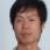0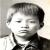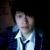0
l

0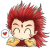0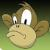0
xx………………这可是有很多错误的。再多的代码没有实用也是废柴呀。